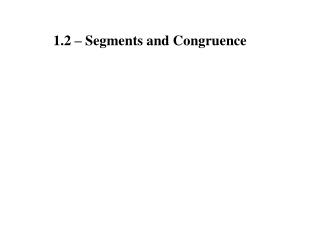DownloadDownload Presentation1.2 – Segments and Congruence

# 1.2 – Segments and Congruence

Télécharger la présentation## 1.2 – Segments and Congruence

- - - - - - - - - - - - - - - - - - - - - - - - - - - E N D - - - - - - - - - - - - - - - - - - - - - - - - - - -
##### Presentation Transcript

1. 1.2 – Segments and Congruence

2. Positive length between two points Segments with the same length A B C D

3. Rules of geometry that are not proved

4. The distance between 2 points is the absolute value of the difference between them. 1 2 3 4 5 6 7 8 7 – 2 = 5u 2 – 7 = 5u

5. Adding two pieces of a segment total the whole B C A AB + BC = AC

6. 1. Measure the length of the segment to the nearest tenth of a centimeter. 3.5 AB = ________cm

7. 1. Measure the length of the segment to the nearest tenth of a centimeter. 1.6 CD = ________cm

8. 1. Measure the length of the segment to the nearest tenth of a centimeter. 2.7 EF = ________cm

9. 2. Find the indicated length. GJ = _______ 16u 16

10. 2. Find the indicated length. ST = _______ 19u 19 37 – 18 =

11. 2. Find the indicated length. 14u YZ = _______ YZ = 3(5) – 1 2x + 3 + 3x – 1 = 27 YZ = 15 – 1 5x + 2 = 27 -2 -2 YZ = 14 5x = 25 x = 5

12. 3. Name the congruent segments using proper notation.

13. 4. Use the number line to find the indicated measure. 4u 5u JK = _____ KL = _____ JL = _____ MK = _____ 9u 10u

14. 5. Plot the given points in a coordinate plane. Then determine whether the line segments named are congruent. A(2, 2), B(2, –1), C(0, –2), D(3, –2) AB = 3u CD = 3u A B C D

15. 5. Plot the given points in a coordinate plane. Then determine whether the line segments named are congruent. E(–3, 2), F(1, 2), G(2, 3), H(2, –2) EF = 4u G GH = 5u E F Not congruent H

16. 6. In the diagram, points P, Q, R, and S are collinear, PS = 46, PR = 18, and PQ = QR. Find the indicated length. PQ = QR = QS = RS = 9u 46 18 9 9u 9 37u 28 28u

17. 7. Point J is between H and K.. Use the given information to draw a diagram and write an equation in terms of x. Solve the equation. Then find HJ and JK. 2x – 5 6x J K H HJ = 2x – 5 JK = 6x 27 HK = 27 HJ + JK = HK HJ = 2x – 5 HJ = 2(4) – 5 2x – 5 + 6x = 27 HJ = 8 – 5 8x – 5 = 27 HJ = 3u +5 +5 8x = 32 JK = 6x 8 8 JK = 6(4) JK = 24u x = 4

18. 7. Point J is between H and K.. Use the given information to draw a diagram and write an equation in terms of x. Solve the equation. Then find HJ and JK. 4x + 7 5x – 8 J K H HJ = 4x + 7 JK = 5x – 8 53 HK = 53 HJ + JK = HK HJ = 4x + 7 HJ = 4(6) + 7 4x + 7 + 5x – 8 = 53 HJ = 24 + 7 9x – 1 = 53 HJ = 31u +1 +1 9x = 54 JK = 5x – 8 9 9 JK = 5(6) – 8 JK = 30 – 8 x = 6 JK = 22u

19. HW Problem # 27 Ans: D Compass, ruler, and protractor, and calculator due Book Cover and Syllabus Due Monday!!!!!!